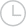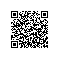## reduceByKey和mapValues如何同时工作？社区小助手2018-12-12 11:13:34283

def parseLine(line):
fields = line.split(',')
age = int(fields)
numFriends = int(fields)
return (age,numFriends)

line = sparkCont.textFile("D:\ResearchInMotion\ml-100k\fakefriends.csv")
rdd = line.map(parseLine)
totalsByAge = rdd.mapValues(lambda x: (x, 1)).reduceByKey(lambda x, y: (x + y, x + y))
averagesByAge = totalsByAge.mapValues(lambda x: x / x)
results = averagesByAge.collect()
for result in results:

``print(result)``取消 提交回答

•社区小助手
2019-07-17 23:20:02

在rdd = line.map(parseLine)你的行中你有一对格式为(age, numFriends)like 的值(a_1, n_1), (a_2, n_2), ..., (a_m, n_m)。在rdd.mapValues(lambda x: (x, 1))你会得到(a_1, (n_1, 1)), (a_2, (n_2, 1)), ..., (a_m, (n_m, 1))。

在reduceByKey，首先按键分组，它意味着所有相同的age组在一个组中，你将有一些喜欢(a_i, iterator over pairs of (n_j, 1) which all n_j has the same age)，然后应用减少的功能。减少部分意味着numFriends每个年龄1彼此相加，并且s彼此相加，1s的总和表示列表中的数字项。

因此，之后reduceByKey，我们将拥有(a_i, (sum of all numFriends in the list, number of items in the list))。换句话说，外部对的第一个值是age，第二个值是内部对，其第一个值是all的numFriends和，第二个值是项目数。因此，totalsByAge.mapValues(lambda x: x / x)给出numFriends了每个人的平均值age。

0 0